#Population DynamicsHere are some natural questions related to population problems:

• What will the population of a certain country be in ten years?
• How are we protecting the resources from extinction?
More can be said about the problem but, in this little review we will not discuss them in detail. In order to illustrate the use of differential equations with regard to this problem we consider the easiest mathematical model offered to govern the population dynamics of a certain species. It is commonly called the exponential model, that is, the rate of change of the population is proportional to the existing population. In other words, if P(t) measures the population, we have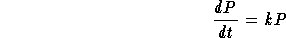,

where the rate k is constant. It is fairly easy to see that if k > 0, we have growth, and if k <0, we have decay. This is a linear equation which solves into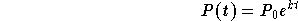,

where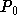is the initial population, i.e.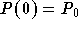. Therefore, we conclude the following:

• if k>0, then the population grows and continues to expand to infinity, that is,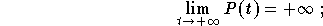• if k<0, then the population will shrink and tend to 0. In other words we are facing extinction.
Clearly, the first case, k>0, is not adequate and the model can be dropped. The main argument for this has to do with environmental limitations. The complication is that population growth is eventually limited by some factor, usually one from among many essential resources. When a population is far from its limits of growth it can grow exponentially. However, when nearing its limits the population size can fluctuate, even chaotically. Another model was proposed to remedy this flaw in the exponential model. It is called the logistic model (also called Verhulst-Pearl model). The differential equation for this model is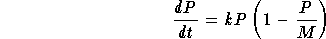,

where M is a limiting size for the population (also called the carrying capacity). Clearly, when P is small compared to M, the equation reduces to the exponential one. In order to solve this equation we recognize a nonlinear equation which is separable. The constant solutions are P=0 and P=M. The non-constant solutions may obtained by separating the variables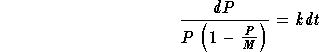,

and integration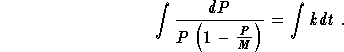The partial fraction techniques gives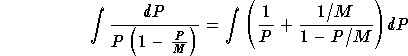,

which gives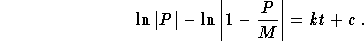Easy algebraic manipulations give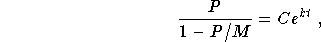where C is a constant. Solving for P, we get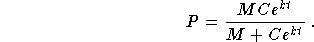If we consider the initial condition(assuming thatis not equal to both 0 or M), we get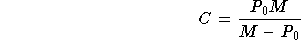,

which, once substituted into the expression for P(t) and simplified, we find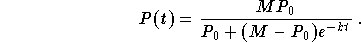It is easy to see that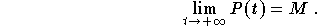However, this is still not satisfactory because this model does not tell us when a population is facing extinction since it never implies that. Even starting with a small population it will always tend to the carrying capacity M.

### If you would like more practice, click on Example.[Differential Equations] [First Order D.E.]
[Geometry] [Algebra] [Trigonometry ]
[Calculus] [Complex Variables] [Matrix Algebra]S.O.S MATHematics home page

Do you need more help? Please post your question on our S.O.S. Mathematics CyberBoard.Author: Mohamed Amine Khamsi